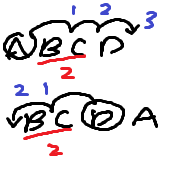### 对排列进行一次对换则改变其奇偶性。

$1\textbf{83}65472 \rightarrow 1\textbf{38}65472$

$13\textbf{86}5472\rightarrow 13\textbf{68}5472$

$1\textbf{38}65472\rightarrow 1\textbf{83}65472$## 全体 n 元排列 $(n>1)$的集合中，奇排列与偶排列各一半

$n>1$ 时，排列数为 $n!$ 并且展开一定会有 $\times2$ 项。因此排列数为偶数。假设奇排列有 o 个，偶排列有 e 个，则有 $o + e = n!$，对于每一个奇排列，取固定的两个位置的数进行对换（比如第一位和最后一位）其将变成偶排列。也就是说可以由此产生 o 个不同偶排列。（为什么这 o 个偶排列各不相同呢？因为假设得到的偶排列相同，那么产生它的奇排列也相同，矛盾。）同理可得可以由此产生 e 个不同奇排列，而 $o + e = n!$，假设 $o \not= e$则，产生的偶排列数 o $\not=$ 全体排列中偶排列的数 e，矛盾。因此必有$o = e$

PS: 其实就是证明由全体奇排列经过一次变换就可以得到全体偶排列，因此必然是各占一半。